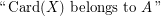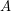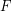# FMT03-Bedlewo

## Monadic second-order logic with cardinality predicates ★★

Author(s): Courcelle

The problem concerns the extension of Monadic Second Order Logic (over a binary relation representing the edge relation) with the following atomic formulas:

\item\itemwhereis a fixed recursive set of integers.

Let us fixand a closed formulain this language.

Conjecture   Is it true that the validity offor a graphof tree-width at mostcan be tested in polynomial time in the size of?

## Fixed-point logic with counting ★★

Author(s): Blass

Question   Can either of the following be expressed in fixed-point logic plus counting:
\item Given a graph, does it have a perfect matching, i.e., a setof edges such that every vertex is incident to exactly one edge from? \item Given a square matrix over a finite field (regarded as a structure in the natural way, as described in [BGS02]), what is its determinant?